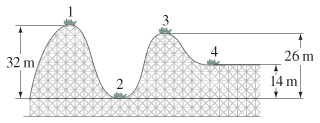# Problem: Suppose the roller-coaster car in the figure passes point 1 with a speed of 3.10 m/s . If the average force of friction is equal to 0.23 of its weight, with what speed will it reach point 2? The distance traveled is 55.0 m .

⚠️Our tutors found the solution shown to be helpful for the problem you're searching for. We don't have the exact solution yet.

###### Problem Details

Suppose the roller-coaster car in the figure passes point 1 with a speed of 3.10 m/s .If the average force of friction is equal to 0.23 of its weight, with what speed will it reach point 2? The distance traveled is 55.0 m .